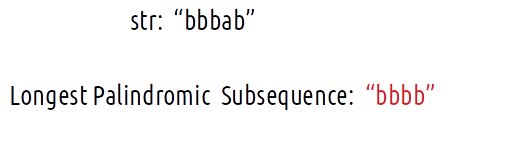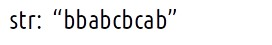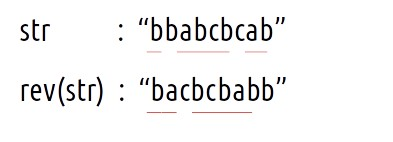# Longest Palindromic Subsequence | (DP-28)

Problem Statement: Longest Palindromic Subsequence

A palindromic string is a string that is equal to its reverse. For example: “nitin” is a palindromic string. Now the question states to find the length of the longest palindromic subsequence of a string. It is that palindromic subsequence of the given string with the greatest length.

```Example:```

There can be many subsequences like “b”, “ba”,”bbb” but “bbbb” is the subsequence that is a palindrome and has the greatest length.

We need to print the length of the longest palindromic subsequence.

Problem Link: Longest Palindromic Subsequence

### Solution :

Approach 1: Using Brute Force

We are given a string S, the simplest approach will be to generate all the subsequences and store them, then manually find out the longest palindromic subsequence.

This naive approach will give us the correct answer but to generate all the subsequences, we will require exponential ( 2n ) time. Therefore we will try some other approaches.

Approach 2: Using Dynamic Programming

Pre-req: Longest Common Subsequence

We can use the approach discussed in the article Longest Common Subsequence, to find the Longest Palindromic Subsequence.

Intuition:

Let us say that we are given the following string.The longest palindromic subsequence will be: “babcbab”.

What is special about this string is that it is palindromic (equal to its reverse) and of the longest length.

Now let us write the reverse of str next to it and please think about the highlighted characters.If we look closely at the highlighted characters, they are nothing but the longest common subsequence of the two strings.

Now, we have taken the reverse of the string for the following two reasons:

• The longest palindromic subsequence being a palindrome will remain the same when the entire string is reversed.
• The length of the palindromic subsequence will also remain the same when the entire string is reversed.

From the above discussion we can conclude:

The longest palindromic subsequence of a string is the longest common subsequence of the given string and its reverse.

Approach:

The algorithm is stated as follows:

• We are given a string (say s), make a copy of it and store it( say string t).
• Reverse the original string s.
• Find the longest common subsequence as discussed in dp-25.

Code:

## C++ Code

``````#include <bits/stdc++.h>

using namespace std;

int lcs(string s1, string s2) {

int n=s1.size();
int m=s2.size();

vector<vector<int>> dp(n+1,vector<int>(m+1,-1));
for(int i=0;i<=n;i++){
dp[i] = 0;
}
for(int i=0;i<=m;i++){
dp[i] = 0;
}

for(int ind1=1;ind1<=n;ind1++){
for(int ind2=1;ind2<=m;ind2++){
if(s1[ind1-1]==s2[ind2-1])
dp[ind1][ind2] = 1 + dp[ind1-1][ind2-1];
else
dp[ind1][ind2] = 0 + max(dp[ind1-1][ind2],dp[ind1][ind2-1]);
}
}

return dp[n][m];
}

int longestPalindromeSubsequence(string s){
string t = s;
reverse(s.begin(),s.end());
return lcs(s,t);
}

int main() {

string s= "bbabcbcab";

cout<<"The Length of Longest Palindromic Subsequence is "<<
longestPalindromeSubsequence(s);
}
``````

Output: The Length of Longest Palindromic Subsequence is 7

Time Complexity: O(N*N)

Reason: There are two nested loops

Space Complexity: O(N*N)

Reason: We are using an external array of size ‘(N*N)’. Stack Space is eliminated.

## Java Code

``````import java.util.*;

class TUF{
static int lcs(String s1, String s2) {

int n=s1.length();
int m=s2.length();

int dp[][]=new int[n+1][m+1];
for(int rows[]:dp)
Arrays.fill(rows,-1);
for(int i=0;i<=n;i++){
dp[i] = 0;
}
for(int i=0;i<=m;i++){
dp[i] = 0;
}

for(int ind1=1;ind1<=n;ind1++){
for(int ind2=1;ind2<=m;ind2++){
if(s1.charAt(ind1-1)==s2.charAt(ind2-1))
dp[ind1][ind2] = 1 + dp[ind1-1][ind2-1];
else
dp[ind1][ind2] = 0 + Math.max(dp[ind1-1][ind2],dp[ind1][ind2-1]);
}
}

return dp[n][m];
}

static int longestPalindromeSubsequence(String s){
String t = s;
String ss=new StringBuilder(s).reverse().toString();
return lcs(ss,t);
}

public static void main(String args[]) {

String s= "bbabcbcab";

System.out.print("The Length of Longest Palindromic Subsequence is ");
System.out.println(longestPalindromeSubsequence(s));
}
}``````

Output: The Length of Longest Palindromic Subsequence is 7

Time Complexity: O(N*N)

Reason: There are two nested loops

Space Complexity: O(N*N)

Reason: We are using an external array of size ‘(N*N)’. Stack Space is eliminated.

### Space Optimization

If we closely we are using two rows: dp[ind1-1][ ], dp[ind][ ],

So we are not required to contain an entire array, we can simply have two rows prev and cur where prev corresponds to dp[ind-1] and cur to dp[ind].

After declaring prev and cur, replace dp[ind-1] to prev and dp[ind] with cur and after the inner loop executes, we will set prev = cur, so that the cur row can serve as prev for the next index.

Code:

## C++ Code

``````#include <bits/stdc++.h>

using namespace std;

int lcs(string s1, string s2) {

int n=s1.size();
int m=s2.size();

vector<int> prev(m+1,0), cur(m+1,0);

// Base Case is covered as we have initialized the prev and cur to 0.

for(int ind1=1;ind1<=n;ind1++){
for(int ind2=1;ind2<=m;ind2++){
if(s1[ind1-1]==s2[ind2-1])
cur[ind2] = 1 + prev[ind2-1];
else
cur[ind2] = 0 + max(prev[ind2],cur[ind2-1]);
}
prev= cur;
}

return prev[m];
}

int longestPalindromeSubsequence(string s){
string t = s;
reverse(s.begin(),s.end());
return lcs(s,t);
}

int main() {

string s= "bbabcbcab";

cout<<"The Length of Longest Palindromic Subsequence is "<<
longestPalindromeSubsequence(s);
}
``````

Output:

The Length of Longest Palindromic Subsequence is 7

Time Complexity: O(N*N)

Reason: There are two nested loops.

Space Complexity: O(N)

Reason: We are using an external array of size ‘N+1’ to store only two rows.

## Java Code

``````import java.util.*;

class TUF{
static int lcs(String s1, String s2) {

int n=s1.length();
int m=s2.length();

int prev[]=new int[m+1];
int cur[]=new int[m+1];

// Base Case is covered as we have initialized the prev and cur to 0.

for(int ind1=1;ind1<=n;ind1++){
for(int ind2=1;ind2<=m;ind2++){
if(s1.charAt(ind1-1)==s2.charAt(ind2-1))
cur[ind2] = 1 + prev[ind2-1];
else
cur[ind2] = 0 + Math.max(prev[ind2],cur[ind2-1]);
}
prev= cur;
}

return prev[m];
}

static int longestPalindromeSubsequence(String s){
String t = s;
String ss=new StringBuilder(s).reverse().toString();
return lcs(ss,t);
}

public static void main(String args[]) {

String s= "bbabcbcab";

System.out.print("The Length of Longest Palindromic Subsequence is ");
System.out.println(longestPalindromeSubsequence(s));
}
}``````

Output:

The Length of Longest Palindromic Subsequence is 7

Time Complexity: O(N*N)

Reason: There are two nested loops.

Space Complexity: O(N)

Reason: We are using an external array of size ‘N+1’ to store only two rows.

Special thanks to Anshuman Sharma for contributing to this article on takeUforward. If you also wish to share your knowledge with the takeUforward fam, please check out this article